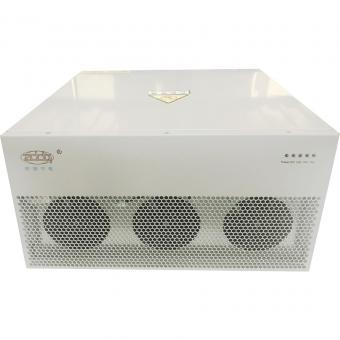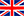English

###### General introduction about harmonics and Causes of harmonics
Dec 25,2019

We make a simple introduction about harmoincs in networks,welcome to communicate with us about harmonics and how to solve.

General Introduction

Harmonics are usually defined by two main characteristics:

• Their amplitude: value of the harmonic voltage or current.
• Their order: value of their frequency with respect to the fundamental frequency (50 Hz).

Under such conditions, the frequency of a 5th order harmonic is five times greater than the fundamental frequency, i.e. 5 x 50 Hz = 250 Hz.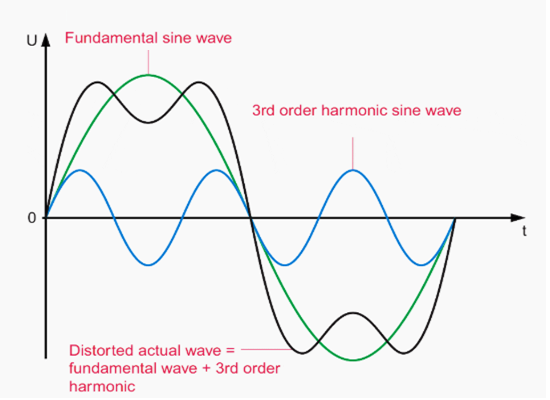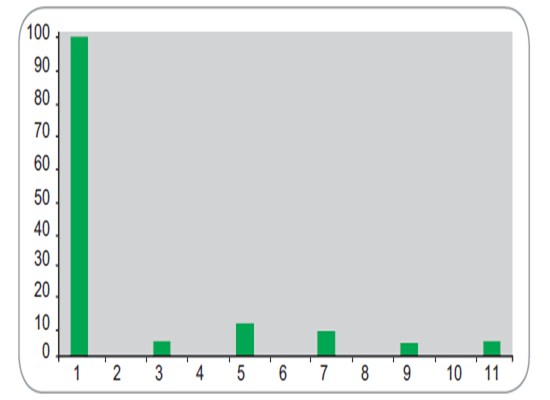Distortion due to a 3rd order harmonics(E.X) Typical graph of the frequency spectrum

Remark:The frequency spectrum, also known as the spectral analysis, indicates the types of harmonic generator present on the network.

The root mean square value

The rms value of a distorted wave is obtained by calculating the quadratic sum of the different values of the wave for all the harmonic orders that exist for this wave:

Rms value of I:

The rms value of all the harmonic components is deduced from this calculation:

This calculation shows one of the main effects of harmonics, i.e. the increased rms current passing through an installation, due to the harmonic components with which a distorted wave is associated.

Usually, the switchgear and cables or the busbar trunking of the installation is defined from the rated current at the fundamental frequency; all these installation components are not designed to withstand excessive harmonic current.

Harmonic measurement: distortion

The presence of varying amounts of harmonics on a network is called distortion.

It is measured by the harmonic distortion rates:

• Th: individual distortion rate

It indicates, as a %, the magnitude of each harmonic with respect to the value of the fundamental frequency:

Th (%) = Ah / A1

Where:

Ah = the value of the voltage or current of the h-order harmonic.

A1 = the value of the voltage or current at the fundamental frequency (50 Hz).

• THD: Total Harmonic Distortion

It indicates, as a %, the magnitude of the total distortion with respect to the fundamental frequency or with respect to the total value of the wave.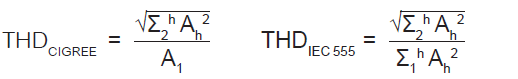The operating values used to find the true situation of the installations with respect to the degree of harmonic contamination are:

The total harmonic voltage distortion [THD(U)] indicating the voltage wave distortion and the ratio of the sum of the harmonic voltages to the fundamental frequency voltage, all expressed as a %.

The total harmonic current distortion [THD(I)] determining the current wave distortion and the ratio of the sum of the harmonic currents to the fundamental frequency current, expressed as a %.

The frequency spectrum (TFT) is a diagram that gives the magnitude of each harmonic according to its order.

By studying it, we can determine which harmonics are present and their

respective magnitude.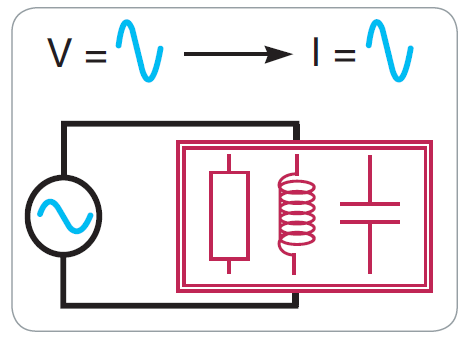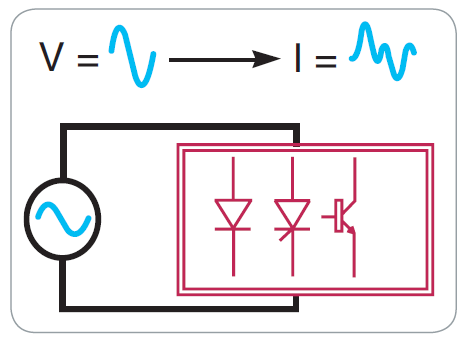Linear loads (inductors, capacitors and resistors) don't generate harmonics. Non-linear loads are those that generate harmonics.

Inter-harmonics

Interharmonics are sinusoidal components with frequencies that are not integral multiples of the fundamental frequency (and therefore situated between the harmonics). They are the result of periodic or random variations of the power absorbed by different loads such as arc furnaces, welding machines and frequency converters (variable speed drives,cycloconvertors).

Causes of Harmonics

Harmonic generators

Harmonics are generally produced by non-linear loads which, although powered by a sinusoidal voltage, absorb a non-sinusoidal current.

In short, non-linear loads are considered to behave as current sources that inject harmonics into the network.

The most common non-linear harmonic loads are those found in devices fed by power electronics, such as variable speed drives, rectifiers, converters, etc.

Loads such as saturable reactors, welding equipment, arc furnaces etc. also inject harmonics.

Other loads have a linear behaviour and do not generate harmonics: inductors, resistors and capacitors.

Main harmonic sources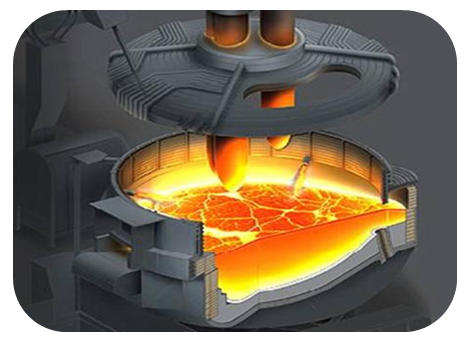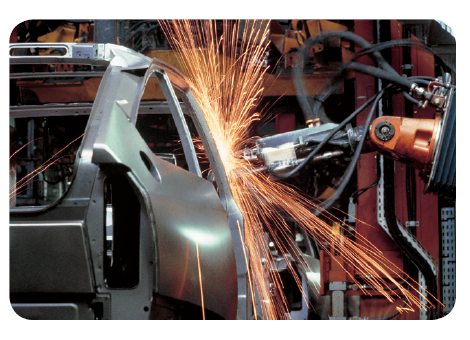Arc Furnace Arc Welding

We differentiate between these loads, according to whether they are used for industrial or residential applications:

a.power electronics devices: variable speed drives, rectifiers, UPS, etc.

b.loads using an electric arc: arc furnaces, welding machines, lighting (fluorescent lamps, etc.); harmonics (temporary) are also generated when motors are started with an electronic starter and when power transformers come into service.

2.Residential loads: TVs, microwave ovens, induction plates, computers, printers, fluorescent lamps, etc.

The following table is a guide to the various loads with information on the injected harmonic current spectrum.

Reference Table of Model Selection for Central Management

Click active harmonic filter for solutions introduction.

Click Active Harmonic Filter Modular for products indroduction.

Some informations refer to ABB and Schneider.

Blog
Hot Products
•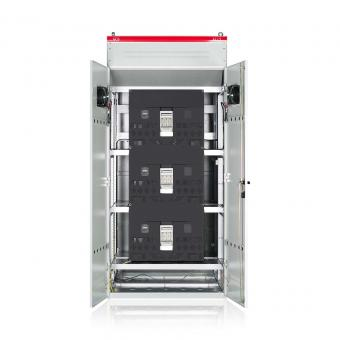#### Capacitor banks and harmonic filters. Low voltage

•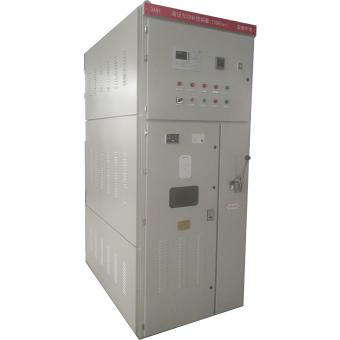#### 3.3KV Local reactive power compensation for motors,Arc Furnace

•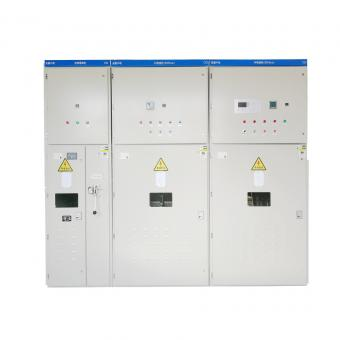#### Medium voltage Automatic Capacitor banks with harmonic filters

•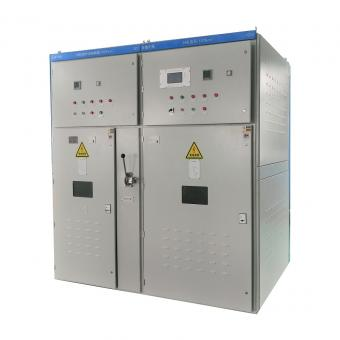#### High voltage Automatic Power Factor improvement plant for Motors VFDs

•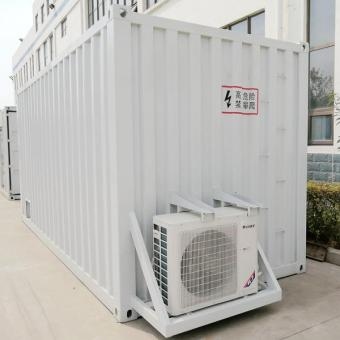#### High voltage static var generator HV SVG Statcom outdoor container

•#### Medium voltage outdoor Static Synchronous Compensator(STATCOM)

•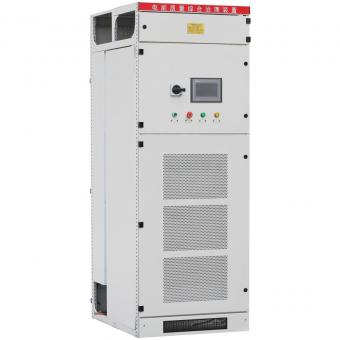•